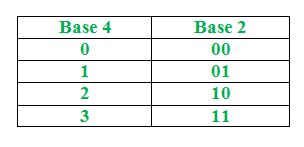# Python program to convert Base 4 system to binary number

• Last Updated : 06 Sep, 2021

Given a base 4 number N, the task is to write a python program to print its binary equivalent.

Conversion Table:Examples:

Input : N=11002233

Output : 101000010101111

Explanation : From that conversion table we changed 1 to 01, 2 to 10 ,3 to 11 ,0 to 00.

Input: N=321321

Output: 111001111001

Approach:

• Take an empty string say resultstr.
• We convert the decimal number to string.
• Traverse the string and convert each character to integer
• If the integer is 1 or 0 then before converting to binary add ‘0’ to resultstr (Because we cannot have 01,00 in integers)
• Now convert this integer to binary string and concatenate the resultant binary string to resultstr.
• Convert resultstr to integer(which removes leading zeros).
• Return resultstr.

Below is the implementation of above approach.

## Python3

 `# function which converts decimal to binary``def` `decToBin(num):``  ` `    ``# Using default binary conversion functions``    ``binary ``=` `bin``(num)``    ` `    ``# removing first two character as the``    ``# result is always in the form 0bXXXXXXX``    ``# by taking characters after index 2``    ``binary ``=` `binary[``2``:]``    ``return` `binary` `# function to convert base4 to binary``def` `convert(num):``  ` `    ``# Taking a empty string``    ``resultstr ``=` `""``    ` `    ``# converting number to string``    ``numstring ``=` `str``(num)``    ` `    ``# Traversing string``    ``for` `i ``in` `numstring:``      ` `        ``# converting this character to integer``        ``i ``=` `int``(i)``        ` `        ``# if i is 1 or 0 then add '0' to result``        ``# string``        ``if``(i ``=``=` `1` `or` `i ``=``=` `0``):``            ``resultstr ``=` `resultstr``+``'0'` `        ``# passing this integer to get converted to``        ``# binary``        ``binary ``=` `decToBin(i)``        ` `        ``# print(binary)``        ``# Concatenating this binary string to result``        ``# string``        ``resultstr ``=` `resultstr``+``binary``        ` `    ``# Converting resultstr to integer``    ``resultstr ``=` `int``(resultstr)``    ` `    ``# Return result string``    ``return` `resultstr`  `# Driver code``Number ``=` `11002233` `# Passing this number to convert function``print``(convert(Number))`

Output:

`101000010101111`

My Personal Notes arrow_drop_up